# C/C++ Preprocessor Metaprogramming - Recursion

Before this, I would recommend reading the Macro Basics Tutorial.  In the article we discussed rule 4 that disallowed recursion explicitly. However, this doesn't mean we cant do recursion. The rule states that if we come across the current macros name it is flagged as a macro that cannot be evaluated. The trick here is that we need an indirect macro that refers to macro we want to recurse. If the indirect macro doesn't evaluate during the macros expansion, we can then evaluate it to recurse. This is hard to follow so lets start start building it up with example. The correct code is at the end (so feel free to skip the derivation) but I want to show where everything comes from.

So first step, lets create an indirection to the recursion macro and see what happens:

#define RECURSE(x) x RECURSE_INDIRECT EMPTY () () (x)
// This the same as #define RECURSE(x) x DEFER(RECURSE_INDIRECT) () (x)
// I just kept the EMPTY() in to make it a bit more explicit

#define RECURSE_INDIRECT() RECURSE
RECURSE(x) // This evaluates to x RECURSE(x)
/*
This example is similar to the mutual recursion example
When the RECURSE macro is expanded, RECURSE_INDIRECT () is also
This causes RECURSE to be added as a result of the initial RECURSE
and as a result we disallow it from expanding more
*/


So the problem is we need to delay the evaluation of the indirection macro so that it is expanded only AFTER the initial macro. How do we do this? We make use of the delay macro discussed before. By adding an EMPTY() we can delay the execution by one step. Lets look at the updated macro

#define EXPR(...) __VA_ARGS__
#define RECURSE(x) x RECURSE_INDIRECT EMPTY () () (x)
#define RECURSE_INDIRECT() RECURSE

RECURSE(x) // This expands to x RECURSE_INDIRECT () (x)!
/*
When we call RECURSE(x) after parameter replacement
[x RECURSE_INDIRECT EMPTY () (x)] is evaluated to
[x RECURSE_INDIRECT(x)]. By adding the EMPTY() we were able to
defer the execution of RECURSION_INDIRECT.
*/

EXPR(RECURSE(x)) // This expands to x x RECURSE_INDIRECT () (x)
/*
We did it!
This is the same as expanding EXPR(x RECURSE_INDIRECT () (x))
Since we expand RECURSION_INDIRECT macro (because it is a paramter to
the EXPR macro), the RECURSE that is added as a result of the expansion
can actually be expanded. This is because RECURSE was a result of
the RECURSION_INDIRECT not the RECURSE macro!

As a result when we evaluate the result of the
expansion RECURSE is evaluated and we have no recursed!.
*/

EXPR(EXPR(RECURSE(x))) // This expands to x x x RECURSE_INDIRECT () (x)


Note, we must make sure the indirection macro doesn't actually call the macro its indirecting explicitly. At which point the indirection would be added as a result of the expansion of the indirection!

#define RECURSE(x) x RECURSE_INDIRECT EMPTY () (x)
#define RECURSE_INDIRECT(x) RECURSE(x)

RECURSE(x) // x RECURSE_INDIRECT (x)

EXPR(RECURSE(x)) // x x RECURSE_INDIRECT (x)
/* Rewrite it as EXPR(x RECURSE_INDIRECT (x)) and we can see we
RECURSE_INDIRECT as a result of expanding RECURSE_INDIRECT
*/

EXPR(EXPR(RECURSE(x))) // As expected we get x x RECURSE_INDIRECT (x)


# Introduction - Basics

First step, the basics. I want to explain how macro expansion works and a couple of rules that we need to keep in mind (Rules 1 and 2 are for beginners while Rules 3 and 4 are worth reviewing). So lets start with a basics and looking at how they work:

#define SIMPLE_DEFINITION REPLACEMENT
#define MACRO(params) STUFF_TO_REPLACE_MACRO_WITH


The first define is a simple replacement. Whenever the compiler comes across SIMPLE_DEFINTION it will replace it with whatever REPLACEMENT does.
The second define is an actual macro. When the compiler comes across MACRO(params) it will replace whatever with whatever we want to replace it with. Macros are evaluated in left to right

RULE 1: Whenever we come across a macro the compiler replaces the macro with whatever the macro text says to replace with. These macros are replaced in left to right order

Rule 2: Macros parameters are directly substituted into the macro (and are evaluated [This is important for the next part]) so watch out for when brackets are needed.

Now lets consider a simple macro definitions and look at how it expands:

#define MULTIPLY(x, y) x * y
MULTIPLY(x, y) // This evaluates to x * y

MULTIPLY(x, MULTIPLY(y, z)) // This evaluates to x * y * z
// The evaluation goes as follows
(1) Expand the first MULTIPLY -> x * MULTIPLY(y, z)
(2) Expand the second MULTIPLY -> x * y * z

MULTIPLY(x + y, z) // This expands to x + y * z not (x + y) * z
MULTIPLY((x + y), z) // This expands to (x + y) * z


Rule 3: During each macro expansion, the parameters are replaced and evaluated

The next one looks how macros are expanded when there is a chain of macros and this is where stuff gets interesting:

#define EMPTY() // This MACRO doesn't do anything
#define EVAL(...) __VA_ARGS__ // This is a simple identity macro
#define HELLO() "Hello World"

HELLO() // Expands to "Hello World" as expected


Now lets complicate stuff and consider what happens in the following example:

HELLO EMPTY() () //  This evaluates to HELLO () not "Hello World"

/*
Lets go over its evaluation

We go left to right and the first macro we can expand is EMPTY()
Note, its not HELLO because we don't actually call it as HELLO ()
and so the compiler just sees it as a normal define not a macro right now.
As a result expanding EMPTY() we end up with
*/
HELLO () // Now note this doesnt expand further
/*
This is because the processor has evaluated all tokens at this point.
How do we get past this problem?
*/


Now, lets look at how to actually evaluate the macro so we end up with "Hello World"

// As the function hints, lets use EVAL and see how it changes our result
EVAL ( HELLO EMPTY() () ) // This evaluates to "Hello World"
/*
The preprocessor first evaluates the EVAL macro.
Now the one thing we need to remember is Rule 2.
The processor puts in the evaluated version of the parameter.
As a result we evaluate the EVAL macro and will output the
evaluated version of HELLO EMPTY() ().
As a result we get the following code
*/
// First pass
HELLO () // Now this will get evaluated as its the result of the EVAL macro
// Send and final pass
"Hello World"


On a side, here's a summary of the rules. Try to see why they would expand the way they do as a result of Rule 3. This is probably one of the most important rules.

HELLO() // "Hello World"
HELLO EMPTY() () // HELLO ()
HELLO EMPTY EMPTY() () () // HELLO EMPTY() ()

EVAL(HELLO EMPTY () ()) // "Hello World"
EVAL(HELLO EMPTY EMPTY() () ()) // HELLO ()
EVAL(EVAL(HELLO EMPTY EMPTY() () ())) // "Hello World"


Rule 4: When a macro is expanded, if the macro name appears during its expansion it is not allowed to be expanded further. HOWEVER, this is only if the macro shows up during its expansion. (This is easier to show then explain)

#define RECURSE(x) x RECURSE(x)
RECURSE(x) // This will evaluate to x RECURSE(x)

EVAL(RECURSE(x)) // This will also still evaluate to x RECURSE(x)
/*
Lets look at a bit more in depth of what happened
EVAL(RECURSE(x)) evaluates to x RECURSE(x)
This is because EVAL causes the RECURSE(x) to evalulate
As a RECURSE(x) expands to x RECURSE(x), the second RECURSE(x)
call is not allowed to be called and stays as RECURSE(x)!
*/


This means no direct mutual recursion allowed either

#define MUTA() a MUTB()
#define MUTB() b MUTA()

MUTA() // This expands to a b MUTA()
/* Lets have a look
(1)   MUTA() expands giving us a MUTB()
(2)   Now we evaluate the expansion and find MUTB().
This expands and gives us a b MUTA(). Now note
this expression is a result of evaluating the MUTA()
expansion. As a result this new MUTA() is not evaluated
(3)   evaluating a b MUTA() simply gives us the same
*/


# FFT Spectral Leakage and Windowing

Spectral leakage happens whenever we introduce components into the frequency spectrum of a signal. First, let's look at this in the case of continuous time with a continuous signal.

Let's say we have a simple sine wave of 2Hz,

Its corresponding fourier transform is an impulse at both positive and negative frequency:

The following plots (assume it extends to infinity in time on both sides) show the signal and its corresponding spectrum: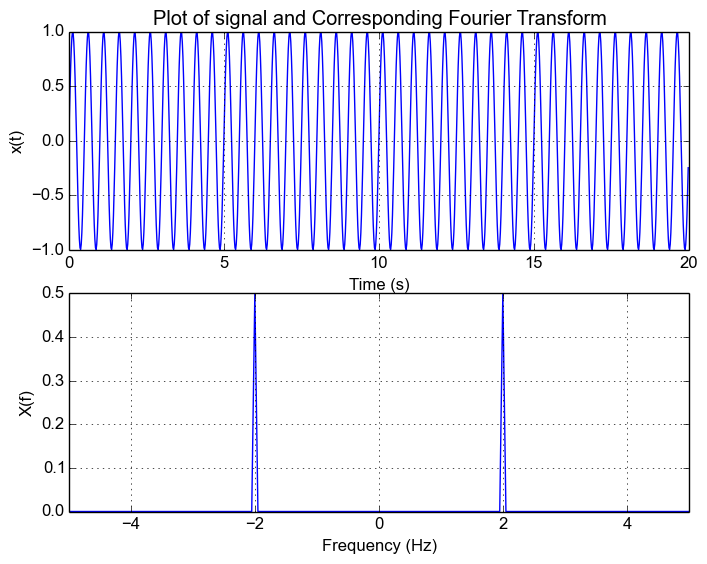## Introducing Windowing

Now, in the case of a real signal, we're only going to have access to a window of the original signal. Let's define this window as . This gives us the signal:

The corresponding Fourier Transform for this new signal is given by the multiplication/convolution theorem

## Spectrum Leakage

Now in the normal case, our window function is a rectangular function. Let's say we sample between  and  and . This window function and corresponding Fourier Transform is

This is exactly what gives rise to spectral leakage. The rectangular window causes the spectrum to now be spread across other frequencies as well.  Going back to the example signal, lets pick a random window from , we get the following responses and we can see the leakage.

Window Response:Windowed Response: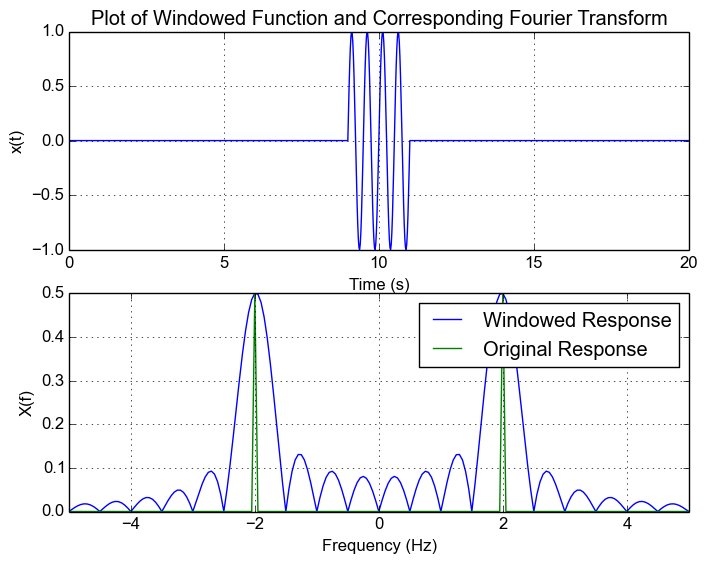Note: In the case were our sample window extends to infinity, our window is . The corresponding Fourier transform of the window . Plugging it back into our expected function for the windowed signal, we have

As a result, we get spikes at both positive and negative frequencies and in what we saw in the first plot.

## Effects of Spectrum Leakage

One of the main issues with spectrum leakage is that the leakage can cause smaller signals to be lost in the leakage caused by another signal.

To show this, let's add use a signal that has a tone at 2Hz and 4Hz:

The following figure shows the response of the result. We can see that we can still make out the 2Hz and 4Hz signal.Now what happens when we scale down the amplitude of the 4Hz signal? We can see from the following figure that this results in the peak of the 4Hz signal not being as visible anymore on top of the leakage.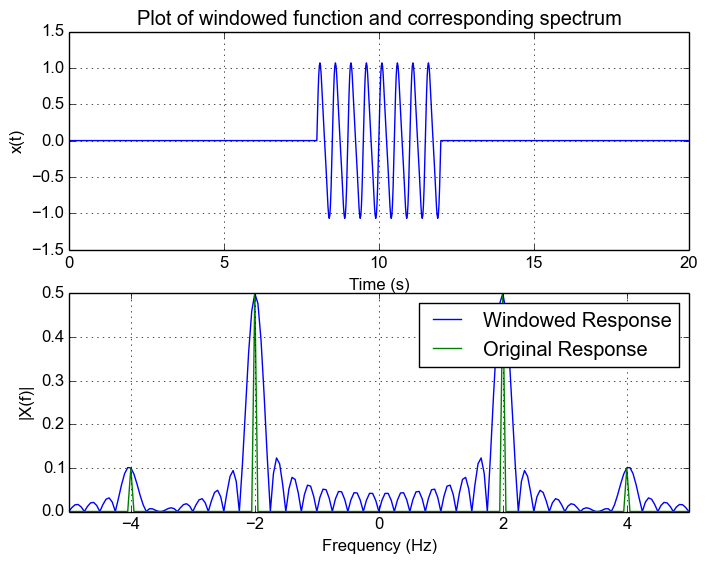Note: In the case of rectangular function, if we increase the number of samples, we reduce the effects of leakage. Furthermore, as a general case, increasing the number of samples is the best way to decrease spectral leakage regardless of what kind of window you use.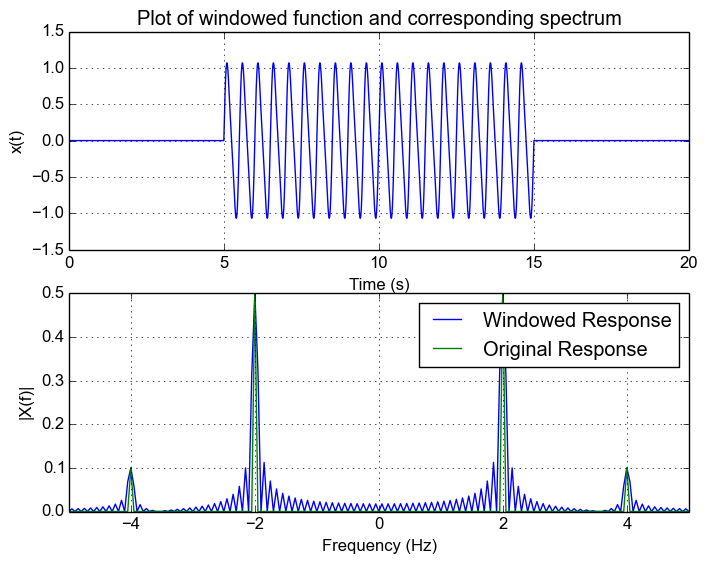## Different Window Functions

Different functions are used in order to reduce how far out the leakage gets. There's a whole list of different functions and their characteristics on this Wikipedia page. I'm not going to go into what the different window functions are and how they affect the result, but the main thing to realize is that the frequency response of the window is convolved with the spectrum of the original signal. There is generally a trade-off between attention of side frequencies and the width of the main lobe.

Let's use the Hanning window and see how it affects our result. For this example, I'm simply replacing the rectangular window with the Hanning window. The following shows the frequency response of the Hanning window. We can see how theres no ringing and it pretty much tapers off. But notice that the width of the main lobe has increased.Now let's apply it to our signal with two frequency components. Note how we can now see the 4Hz signal! This is what using different windowing functions lets us achieve.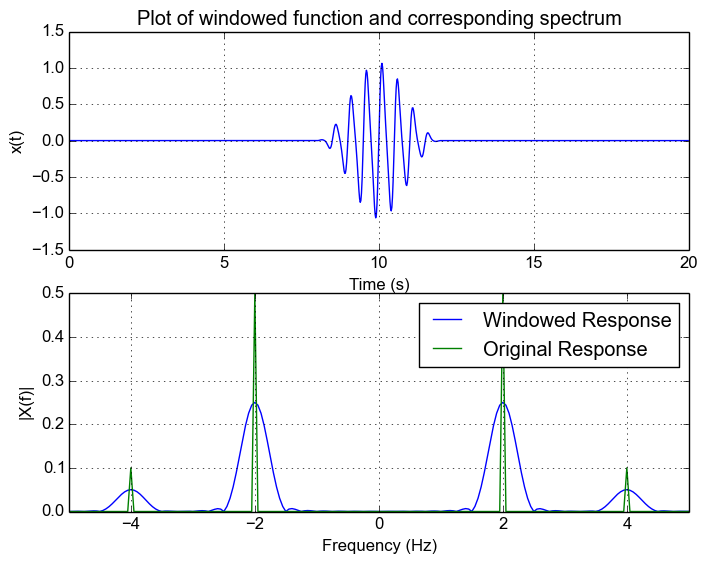## FFT/Discrete Fourier Transform

Now, in the real world we only have a discretely sampled signal. This doesn't affect our result very much. The real main difference we see is that the FFT essentially samples the frequency response, and that our frequency range is now limited to the sample rate.

Let's say we now sample our original 2Hz signal and sample at 10Hz for 6 cycles (this is actually how I generated the initial plot), we can see that we get the same frequency response as in the original example. This is because at sampled frequencies of the rectangular window frequency response, the sampled values are zero. This is because The FFT essentially obtains a sampled version of the continuous response which we can see in the following plot.

Actually, if you zero-pad the signal you will actually see the FFT no longer looks like a spike and instead you will see the sinc response.This is because by zero padding your are increasing the resolution of the FFT and you will sample at non-integer points and see the behaviour.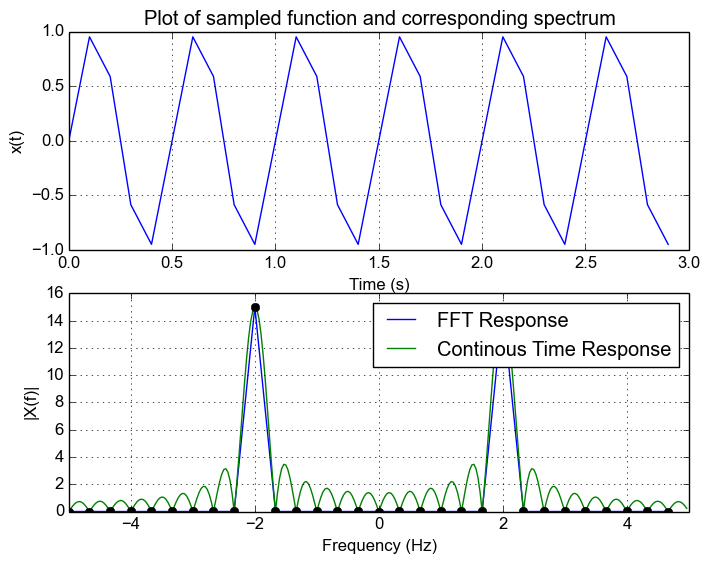Now what happens when we don't have an integer number of samples? Let's say we sample for 6 cycles plus 1 sample. We can see that our FFT looks spread out. The underlying reason is that we're no longer sampling at the zero points in the freuqnecy response of the window and are now sampling part of the leakage caused by a rectangle function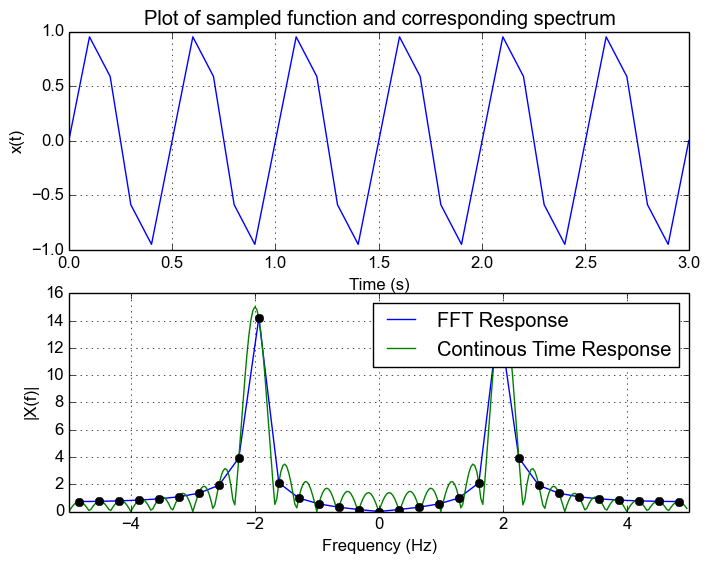Now let's use the Hanning window. We can see that the leakage is reduced and FFT now looks more like a spike and is contained to a couple of nearby bins.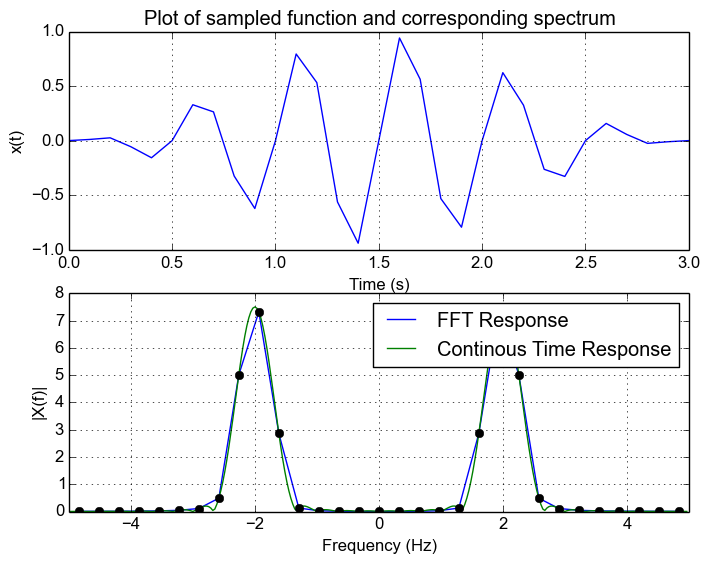## Conclusion

Spectrum leakage happens because of the window we use on our signal when we sample it. The window functions frequency response is convolved with the frequency response of the original signal. This window function introduces spectral leakage and the effect of the leakage depends on the window function.

For a discrete signal with appropriate sample rate such that there is no aliasing, the FFT is simply a discretely sampled continuous version of the  band limited spectrum. As a result, leakage will occur when an FFT is computed of a signal sampled of a non-integer number of cycles.

Choosing an appropriate window will help get rid of this leakage and care must be chosen to see how it affects the spectrum.